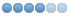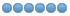# Changing the default display

• Nero
•Topic Author
• Offline
•4 years 10 months ago #8247 by Nero
Changing the default display was created by Nero
Hello fellow builders,

I am wanting to update the display on my mud

Currently it displays like this < 309H 493M 264V > but I want it to be more advanced and have it to where it also display more like:

Opponent: / Tank:
2147(2147)Hitpoints 638(638)Mana 261(261)Move>

I want it to display the Opponent(mob) you, or your group, is facing and who the current tank of that Opponent(mob) is.
I have the current code for the setup I have now but not sure exactly how to convert it to what I want. Any help would be much appreciated.

```char *make_prompt(struct descriptor_data *d)
{
static char prompt[MAX_PROMPT_LENGTH];
char_data *ch = d->character;

/* Note, prompt is truncated at MAX_PROMPT_LENGTH chars (structs.h) */

if (d->showstr_count) {
snprintf(prompt, sizeof(prompt),
"\r\n[ Return to continue, (q)uit, (r)efresh, (b)ack, or page number (%d/%d) ]",
d->showstr_page, d->showstr_count);
} else if (d->str)
strcpy(prompt, "] ");
else if (STATE(d) == CON_PLAYING && !IS_NPC(ch)) {
int count=0;
static char diagnosis[MAX_PROMPT_LENGTH] = "";
size_t len = 0;

*prompt = '\0';
if ((PRF_FLAGGED(ch, PRF_DISPHP) || PRF_FLAGGED(ch, PRF_DISPMANA) || PRF_FLAGGED(ch, PRF_DISPMOVE) || PRF_FLAGGED(ch, PRF_DISPEXP) || PRF_FLAGGED(ch, PRF_DISPTARGET) || PRF_FLAGGED(ch, PRF_DISPAUTO)) && len < sizeof(prompt)) {
count = snprintf(prompt + len, sizeof(prompt) - len, "@W<@n ");
if (count > 0)
len += count;
}
if (GET_INVIS_LEV(ch) && len < sizeof(prompt)) {
count = snprintf(prompt + len, sizeof(prompt) - len, "i%d ", GET_INVIS_LEV(ch));
if (count > 0)
len += count;
}
/* show only when below 25% */
if (PRF_FLAGGED(ch, PRF_DISPAUTO) && len < sizeof(prompt)) {
if (GET_HIT(ch) << 2 < GET_MAX_HIT(ch) ) {
if (count > 0)
len += count;
count = snprintf(prompt + len, sizeof(prompt) - len, "@R%dH@n ", GET_HIT(ch));
if (count > 0)
len += count;
}
if (GET_MANA(ch) << 2 < GET_MAX_MANA(ch) && len < sizeof(prompt)) {
count = snprintf(prompt + len, sizeof(prompt) - len, "@G%dM@n ", GET_MANA(ch));
if (count > 0)
len += count;
}
if (GET_MOVE(ch) << 2 < GET_MAX_MOVE(ch) && len < sizeof(prompt)) {
count = snprintf(prompt + len, sizeof(prompt) - len, "@Y%dV@n ", GET_MOVE(ch));
if (count > 0)
len += count;
}
if ((len < sizeof(prompt)) && (GET_LEVEL(ch) < LVL_IMMORT))
{
count = snprintf(prompt + len, sizeof(prompt) - len, "@C%dX@n ", get_xp_needed(ch));
if (count > 0)
len += count;
}
if (len < sizeof(prompt)) {
get_opponent_diagnosis(ch, diagnosis);
count = sizeof(diagnosis);
if (count > 0)
len += count;
strncat(prompt,diagnosis, sizeof(prompt) - len - 1);
}
}
else
{ /* not auto prompt */
if (PRF_FLAGGED(ch, PRF_DISPHP) && len < sizeof(prompt)) {
count = snprintf(prompt + len, sizeof(prompt) - len, "@R%dH@n ", GET_HIT(ch));
if (count > 0)
len += count;
}

if (PRF_FLAGGED(ch, PRF_DISPMANA) && len < sizeof(prompt)) {
count = snprintf(prompt + len, sizeof(prompt) - len, "@G%dM@n ", GET_MANA(ch));
if (count > 0)
len += count;
}

if (PRF_FLAGGED(ch, PRF_DISPMOVE) && len < sizeof(prompt)) {
count = snprintf(prompt + len, sizeof(prompt) - len, "@Y%dV@n ", GET_MOVE(ch));
if (count > 0)
len += count;
}

if ((GET_MANA_SHIELD(ch) >= 1) && len < sizeof(prompt)) {
count = snprintf(prompt + len, sizeof(prompt) - len, "@W%dS@n ", GET_MANA_SHIELD(ch));
if (count > 0)
len += count;
}

if (PRF_FLAGGED(ch, PRF_DISPEXP) && (len < sizeof(prompt)) && (GET_LEVEL(ch) < LVL_IMMORT)) {
count = snprintf(prompt + len, sizeof(prompt) - len, "@C%dX@n ", get_xp_needed(ch));
if (count > 0)
len += count;
}
if (PRF_FLAGGED(ch, PRF_DISPTARGET) && len < sizeof(prompt)) {
get_opponent_diagnosis(ch, diagnosis);
count = sizeof(diagnosis);
if (count > 0)
len += count;
strncat(prompt,diagnosis, sizeof(prompt) - len - 1);
}
if (PRF_FLAGGED(ch, PRF_BUILDWALK) && len < sizeof(prompt)) {
count = snprintf(prompt + len, sizeof(prompt) - len, "BUILDWALKING ");
if (count >= 0)
len += count;
}
}
//if (len < sizeof(prompt))
strncat(prompt, "@W>@n ", sizeof(prompt) - len - 1);	/* strncat: OK */
proc_colors(prompt, sizeof(prompt), COLOR_ON(ch));
}
else if (STATE(d) == CON_PLAYING && IS_NPC(ch))
snprintf(prompt, sizeof(prompt), "%s> ", GET_NAME(ch));
else
*prompt = '\0';
return (prompt);
}```

• WhiskyTest
•• Offline
• Platinum Member
•4 years 10 months ago #8248 by WhiskyTest
Replied by WhiskyTest on topic Changing the default display
Here's one I prepared earlier which may be of interest:

tbamud.com/forum/8-snippets/4404-bespoke-prompt

• Nero
•Topic Author
• Offline
•4 years 9 months ago #8250 by Nero
Replied by Nero on topic Changing the default display
With a few tweaks, this might be exactly what I am looking for. Thanks for sharing!

• Parnassus
• Offline
•